Next: Problems Up: Alternating Current Circuits Previous: Four-Terminal Networks

# Single-Term Approximations of H

A circuit with a few components quickly leads to a complicated expression for the transfer function. It is often sufficient, and of course, easier to work with approximations to the transfer function.

Let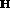be the ratio of two polynomials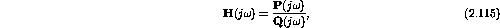where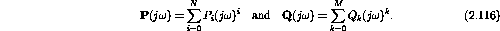If one term dominates in each polynomial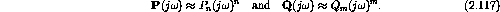Thus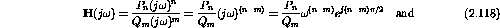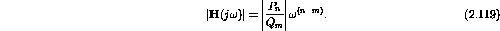We define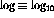and plot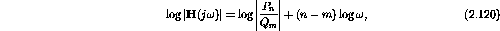which is a straight line on a log-log plot with integer slope.

As an example, consider our RCL circuit:where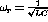and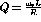.

At low frequencies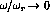and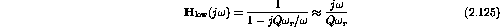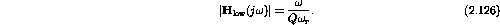On a log-log plot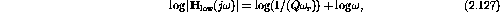which has a slope of +1.

At high frequencies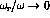and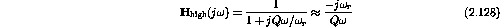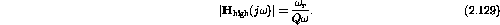On a log-log plot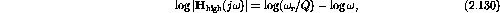which has a slope of -1.

Doug Gingrich
Tue Jul 13 16:55:15 EDT 1999